## Counting in multiples of 6, 7, 8 and 9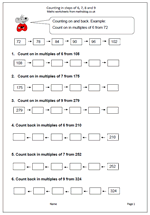A new target in the Maths Primary Framework for Year 4 is to count up in steps of 6, 7, and 9. This might sound easy and if the numbers are low there should not be a problem. However, counting up and down in steps of 6 or 7 starting from larger numbers can be tricky and might well involve a great deal of finger counting. Don’t be surprised at this as there is a lot of mathematical knowledge needed to successfully and quickly count up 7 from a number such as 196. It can be done by counting in single digits, or counting 4 to 200 and then 3, or even adding 10 and subtracting 3.

Counting in steps of 6,7,8 and 9

## Subtracting mentally in Year 4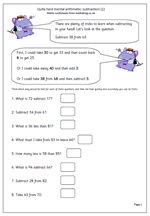Making the correct choice in deciding how to work out an answer is the key to fast mental arithmetic. Many children are unaware that there are often several ways of working out a calculation and that those who choose the best methods find maths easier and are able to answer questions more quickly and more accurately.

Let’s look at ‘Subtract 38 from 63.

This should be done, ‘in your head’ and will involve several stages, depending on the method used.

First, I could take 30 from 63 to leave 33. Then I could count back 8 from 33 which gives me 25.

Secondly, I could take 40 from 63 leaving 23 and then compensate by adding 2, which gives me 25.

Thirdly, I could take 38 from 68, leaving 30 and then compensate by subtracting 5 (because 63 is 5 less than 68).

There are several other ways very similar to these, but this does show that there is no ‘one right way, when it comes to mental arithmetic.

Quite hard mental arithmetic:_subtraction (1)

## Revising times tables in Year 4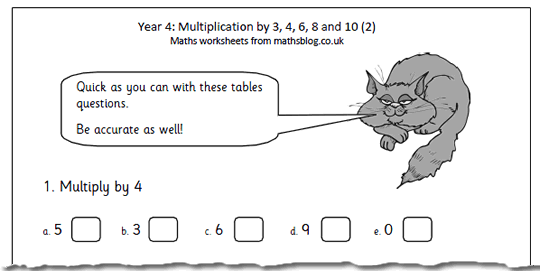What have we got today? It’s the second in our multiplying by 3, 4, 6, 8 and 10 worksheets, suitable for year 4 children or those who are beginning to know their tables.

The best way to learn tables is to recite them out loud eg ‘4 times 3 is 12, then shorten it to,  ‘4 3s are 12’, with the ultimate aim of being able to say the product for any two single digits without having to work it out.

During the process of learning times tables plenty of practice is needed and how well they have been learned can be seen by how quickly the page is correctly answered.

Multiplication 3x 4x 6x 8x 10_(pg 2)

## Subtracting from whole thousands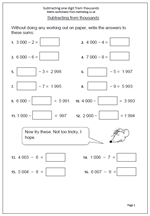Sometimes it is much easier to carry out a calculation mentally (in your head) than to use the standard written method. This page of questions illustrates this perfectly. It looks at taking a one-digit number away from a multiple of a thousand.

if this was written down on paper it would involve considerable amounts of crossing out and ‘borrowing’ from thousands to hundreds and then from hundreds to tens; a potential minefield for children. However, if they just count back from the whole thousands, even using fingers to check that they have counted back the correct number, hey presto, the answer is found in seconds.

Similarly, with a question such as 4 000 – ??? = 3993 adding on can be employed: start with 3993 and counting on until 4 000 is reached. Simple.

Subtract a single digit from thousands

## Year 4 maths worksheet: decimal fractions and tenths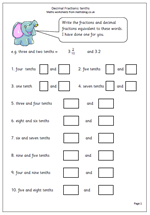Some children do not realise that a fraction such as 2/10 can also be written as a decimal i.e. 0.2. In one sentence we have two of the major sticking points in maths, but here we have a maths worksheet which neatly combines the two, showing the equivalence between fractions and decimal fractions.

One way to show this is to use the fraction as a division sum. 2/10 can be seen as 2 divided by 10. Do this on a calculator to get 0.2. Also remember, to divide by ten mentally, just move each digit one place to the right, putting in the decimal point if moving from units to tenths. This page can be found in our year 4 resources.

Decimal_fractions_tenths_(2)

## Year 4: What sign?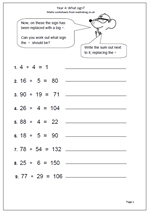Children in Year 4 will be investigating a whole range of problems involving number and being able to recognise and explain patterns. Children should then be able to extend the ideas presented and use these to make predictions and ask ‘What if….?’ questions.

Problems may appear in many forms such as the following:

Find numbers that satisfy a particular relationship such as totalling a given number.

Adding operations of addition, subtraction, multiplication and division to a given set of numbers to make a given answer.

Fill in missing digits or missing signs.

This page looks at finding missing signs. The calculations probably don’t need to be worked out, especially if the child has a ‘feel’ for numbers.

A good extension of this is to have three or four number cards and signs and see what whole number answers can be made.

What sign?

## Resource of the week: adding 2-digit numbers mentally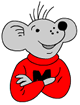One of the keys to success with maths is being able to calculate mentally. There should be no need to resort to written methods or to a calculator to add two 2-digit numbers; mental calculations should rule!

Having said that, these are not easy, and a number of skills need to have been learned to do these in an efficient manner.

For example: looking at ‘add 46 to 67’.

There a a number of ways to do this. Perhaps the most efficient is to add 40 to 67 to make 107 and then add on the extra 6 to make 113.
The second part of the sheet is a reminder of some of the ways that addition questions can be phrased.

## Reading and writing larger numbers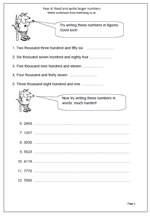In Year 4 children are expected to be working with larger numbers, including thousands. However, it is often presumed that they can read and write these numbers without any practice. This is not the case, as many children find difficulty with this and often demonstrate the problem when asked to write down a number such as six thousand and five. They will write:

60005

What they have done here is write the whole of the 6000 and then added the 5 on at the end rather than 6 005.

When reading numbers it is best to start working them out from the first three numbers on the right  and block the numbers into hundreds, tens and units. Then do the same with the next three, remembering that they are hundreds tens and units of thousands – these even bigger numbers will come into Year 5.

It is a good idea to separate each block of HTU in some way: a popular convention in the UK is to use a comma or a small space.

## Resource of the Week: Easter co-ordinates for Year 4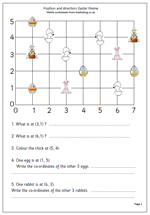As we move towards Easter it is always fun to have some maths linked to the time of year.

There are several important aspects to this page. Firstly the axes are numbered rather than the spaces. This is an important step as the conventions of using co-ordinates come into play. It is important to show how co-ordinates are written:

e.g. (3, 1)

Brackets are always placed around the co-ordinates, with the numbers separated by a comma. The position (3, 1) means 3 along and 1 up, which in this case takes you to the pink rabbit (and not the rabbit holding the basket of eggs).

The grid can be used for further work: e.g.

1.ask how you can move along the lines to go from one point to another (2 along and 1 up)

2. Draw more features at the points and ask where they are positioned.

3. Ask child to draw an egg at a certain point etc.

This can be found in the Year 4 Shape category.

Thanks to urbrainy.com for allowing me to use it.

Easter coordinates

## Resource of the week: Money and place value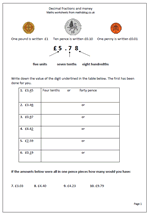Money is a great way to reinforce place value with decimals. For example in the total £3.45 the digit 4 has a value of 4 tenths or 40p. Money makes it very easy to show tenths (a 10p coin is one tenth of a pound) and hundredths (a one pence coin is one hundredth of a pound).

This page looks at this in more detail, showing that ten pence can be written in two ways; either as 10p or as part of a pound £0.10. There are two further things to remember here:

1. that we should always have two digits after the decimal point when writing fractions of a pound eg £0.30 not £0.3.

2. that we should not include both the pound and pence sign when writing amounts in pounds eg £0.30 is correct but £0.30p is not. it is 0.30 of a pound, not 0.30 of a penny.

This is just one worksheet from a great selection in our Counting and Understanding Numbers section within our year 4 maths worksheets. Why not go and have a look at what else is freely available?

Decimal fractions and money